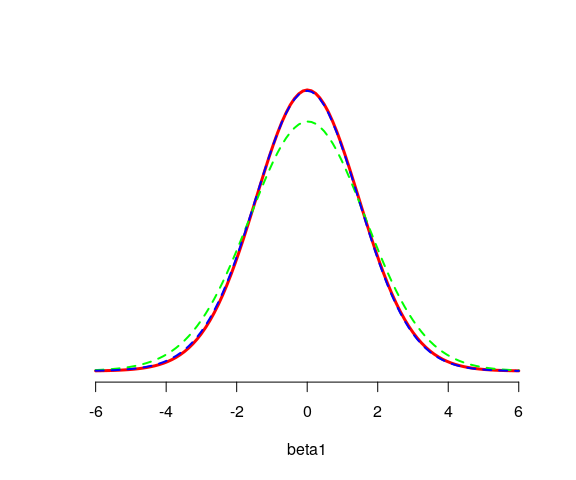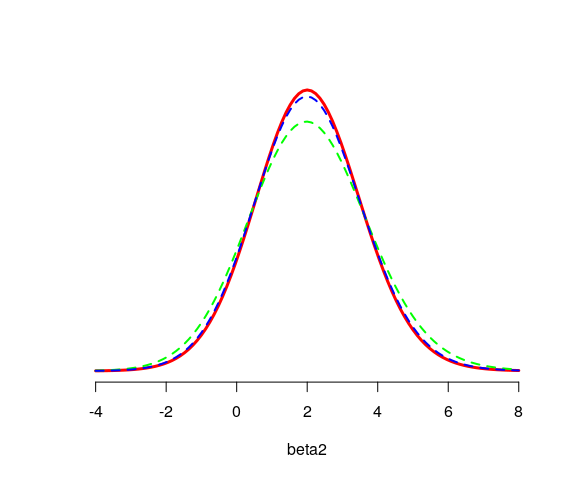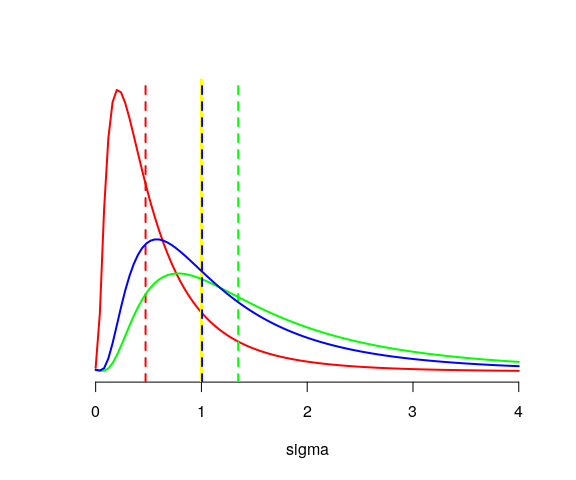# The ‘gfilinreg’ package

The main function of the ‘gfilinreg’ package, namely gfilinreg, returns some weighted samples of the generalized fiducial distribution of the parameters of a linear regression model whose error terms are distributed according to a Gaussian, Student, Cauchy, or a logistic distribution.

Let’s have a look at a simple linear regression model with Gaussian errors:

library(gfilinreg)
set.seed(666L)
x <- rgamma(6L, shape = 10, rate = 1)
y <- rnorm(6L, mean = x, sd = 2)
dat <- data.frame(x = x, y = y)
fidsamples <-
gfilinreg(y ~ x, data = dat, distr = "normal", L = 100L, nthreads = 2L)

The algorithm involves a partition of the hypercube $${(0,1)}^{p+1}$$, where $$p$$ is the dimension of the model (the number of columns of the $$X$$ matrix), and the integer argument L of the gfilinreg function is the desired number of subdivisions of each edge of the hypercube.

Note. I set nthreads = 2L because of CRAN constraints: CRAN does not allow more than two parallel computations. This is also why I set nthreads = 2L in all the examples of the package.

A quick summary of the fiducial samples is provided by the gfiSummary function:

gfiSummary(fidsamples)
#>                  mean    median        lwr      upr
#> (Intercept) -2.914704 -2.913264 -8.7942860 2.959696
#> x            1.288755  1.287826  0.7684114 1.811606
#> sigma        1.965359  1.706877  0.9922482 4.476055
#> attr(,"confidence level")
#>  0.95

Let’s compare with lm:

lmfit <- lm(y ~ x, data = dat)
coefficients(lmfit)
#> (Intercept)           x
#>   -2.916874    1.289811
sigma(lmfit)
#>  1.551726
confint(lmfit)
#>                 2.5 %   97.5 %
#> (Intercept) -8.757421 2.923674
#> x            0.771654 1.807969

Excepted for the scale parameter $$\sigma$$, results are very similar.

One can generate simulations of fiducial predictive distributions and, based on these distributions, one can get fiducial prediction intervals:

new <- data.frame(x = c(9, 10, 11))
fidpred <- gfilinregPredictive(fidsamples, newdata = new)
gfiSummary(fidpred)
#>         mean    median      lwr      upr
#> y1  8.682954  8.674565 3.925779 13.46422
#> y2  9.970281  9.964336 5.287119 14.68178
#> y3 11.261375 11.253485 6.590240 15.96771
#> attr(,"confidence level")
#>  0.95
predict(lmfit, newdata = new, interval = "prediction")
#>         fit      lwr      upr
#> 1  8.691428 3.950576 13.43228
#> 2  9.981240 5.311629 14.65085
#> 3 11.271051 6.615751 15.92635

Again, there is a strong agreement between the fiducial results and the frequentist results.

## A small simulation study with Cauchy errors

Now we perform some simulations of a “t-test model” with Cauchy errors, we store the fiducial summaries for each simulated dataset and we also store the maximum likelihood estimates thanks to the ‘heavy’ package. We simulate $$1000$$ datasets.

library(gfilinreg)
library(heavy)
library(data.table)

nsims <- 1000L
MAXLHD <- matrix(NA_real_, nrow = nsims, ncol = 3L)
colnames(MAXLHD) <- c("group1", "group2", "sigma")
FIDlist <- vector("list", length = nsims)

group <- gl(2L, 3L)
set.seed(666L)

for(i in 1L:nsims){
# simulated dataset
dat <- data.frame(
group = group,
y = c(rcauchy(3L), 2 + rcauchy(3L))
)
# max-likelihood estimates
hfit <- heavyLm(y ~ 0 + group, data = dat, family = Cauchy())
MAXLHD[i, ] <- c(hfit[["coefficients"]], sqrt(hfit[["sigma2"]]))
# fiducial stuff
fidsamples <- gfilinreg(y ~ 0 + group, data = dat, L = 100L, distr = "cauchy")
FIDlist[[i]] <- cbind(
parameter = c("group1", "group2", "sigma"),
as.data.table(gfiSummary(fidsamples))
)
}
FID <- rbindlist(FIDlist)

The above simulations are not performed in this vignette. They are available in the package:

library(data.table)
data("FID")
data("MAXLHD")

### Estimates

Our three estimates of the group1 parameter (maximum likelihood, fiducial mean, fiducial median) have a similar distribution:

library(kde1d)
group1_maxlhd     <- MAXLHD[, "group1"]
group1_fid_mean   <- FID[parameter == "group1"][["mean"]]
group1_fid_median <- FID[parameter == "group1"][["median"]]

kfit_maxlhd     <- kde1d(group1_maxlhd, mult = 4)
kfit_fid_mean   <- kde1d(group1_fid_mean, mult = 4)
kfit_fid_median <- kde1d(group1_fid_median, mult = 4)

curve(
dkde1d(x, kfit_maxlhd), from = -6, to = 6, axes = FALSE,
lwd = 3, col = "red", xlab = "beta1", ylab = NA
)
axis(1)
curve(
dkde1d(x, kfit_fid_mean), add = TRUE,
lwd = 2, col = "green", lty = "dashed"
)
curve(
dkde1d(x, kfit_fid_median), add = TRUE,
lwd = 2, col = "blue", lty = "dashed"
)The distributions of the three estimates of the group2 parameter are similar as well:

group2_maxlhd     <- MAXLHD[, "group2"]
group2_fid_mean   <- FID[parameter == "group2"][["mean"]]
group2_fid_median <- FID[parameter == "group2"][["median"]]

kfit_maxlhd     <- kde1d(group2_maxlhd, mult = 4)
kfit_fid_mean   <- kde1d(group2_fid_mean, mult = 4)
kfit_fid_median <- kde1d(group2_fid_median, mult = 4)

curve(
dkde1d(x, kfit_maxlhd), from = -4, to = 8, axes = FALSE,
lwd = 3, col = "red", xlab = "beta2", ylab = NA
)
axis(1)
curve(
dkde1d(x, kfit_fid_mean), add = TRUE,
lwd = 2, col = "green", lty = "dashed"
)
curve(
dkde1d(x, kfit_fid_median), add = TRUE,
lwd = 2, col = "blue", lty = "dashed"
)For the scale parameter sigma, there are some differences. The maximum likelihood estimates often underestimate the true value, and the true value is close to the median of the fiducial medians:

sigma_maxlhd     <- MAXLHD[, "sigma"]
sigma_fid_mean   <- FID[parameter == "sigma"][["mean"]]
sigma_fid_median <- FID[parameter == "sigma"][["median"]]

kfit_maxlhd     <- kde1d(sigma_maxlhd, xmin = 0, mult = 4)
kfit_fid_mean   <- kde1d(sigma_fid_mean, xmin = 0, mult = 4)
kfit_fid_median <- kde1d(sigma_fid_median, xmin = 0, mult = 4)

curve(
dkde1d(x, kfit_maxlhd), from = 0, to = 4, axes = FALSE,
lwd = 2, col = "red", xlab = "sigma", ylab = NA
)
axis(1)
abline(v = median(sigma_maxlhd), col = "red", lwd = 2, lty = "dashed")
abline(v = 1, col = "yellow", lwd = 3) # true value
curve(
dkde1d(x, kfit_fid_mean), add = TRUE,
lwd = 2, col = "green"
)
abline(v = median(sigma_fid_mean), col = "green", lwd = 2, lty = "dashed")
curve(
dkde1d(x, kfit_fid_median), add = TRUE,
lwd = 2, col = "blue"
)
abline(v = median(sigma_fid_median), col = "blue", lwd = 2, lty = "dashed")### Frequentist coverage

Below are the coverage probabilities of the fiducial confidence intervals estimated from the $$500$$ simulations.

# group1
group1 <- FID[parameter == "group1"]
mean(group1[["lwr"]] < 0)
#>  0.978
mean(0 < group1[["upr"]])
#>  0.979
mean(group1[["lwr"]] < 0 & 0 < group1[["upr"]])
#>  0.957
# group2
group2 <- FID[parameter == "group2"]
mean(group2[["lwr"]] < 2)
#>  0.982
mean(2 < group2[["upr"]])
#>  0.977
mean(group2[["lwr"]] < 2 & 2 < group2[["upr"]])
#>  0.959
# sigma
sigma <- FID[parameter == "sigma"]
mean(sigma[["lwr"]] < 1)
#>  0.921
mean(1 < sigma[["upr"]])
#>  0.986
mean(sigma[["lwr"]] < 1 & 1 < sigma[["upr"]])
#>  0.907

For the parameters group1 and group2, the coverage probabilities are close to the nominal level. For sigma, only the upper bound yields a coverage probability close to the nominal level. The lower bound is too large on average. But remember that the datasets we simulated are small ($$3$$ observations per group).